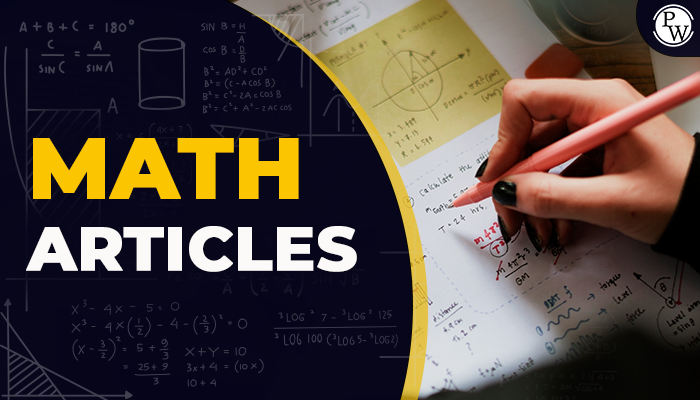# Math Articles

Mathematics is the queen of science, and arithmetic is the queen of mathematics" - by Carl Fredrich Gauss

Mathematics is the fundamental science that helps to understand the scientific and technical fields. Not only in terms of scientific and technical areas but also essential in real life as well. From basic calculations to currency flow, mathematics is used in everything.That’s why today we’re going to explain the mathematics articles which carry almost all the topics covered in the school. The articles will cover everything from the basic to the advanced level, enlightening your knowledge and converting it into a structured form.

You can also check the reference list during the examination to revise and understand all the topics given in the textbook.

## What are the 7 Strands of Mathematics?

There’re 7 mathematical strands in the mathematics subject named as:

1. Numbers
2. Operations
3. Data handling
4. Relations & Functions
5. Measurements
6. Ratio
7. Geometry

These strands are explained in every grade but according to the level of the students. These strands help you discover the problems and look at the world through a bigger picture.

Check out the complete list of all the mathematical articles with all the links mentioned below:

 List of All Math Articles Trigonometry Formula 1 Billion in Rupees Composite Numbers Greater Than and Less Than Symbols Liters to Milliliters Number Names 1 to 100 Successor and Predecessor Trillion Feet to cm Value of Pi Algebraic Identities Exponents Average Cost Associative Law Co-Prime Numbers Cylinder Common Factors Straight Angle Ratio to Percentage Profit and Loss Bisector

### How are Maths Formula important for Students?

Students often underestimate the need for mathematics formulas. Here’s why maths formulas are essential for students.

1. They’re essential to understanding any concept mentioned in the textbooks.
2. With Maths Formulas, you can also understand the fundamental concepts of life.
3. You can solve the equations in an easier and faster way.
4. Sometimes, maths formulas can give you the answers to complicated equations in one go.
5. It is used to state the relationship between the quantities.
6. They’re also helpful for the students preparing for the competitive examination.

### What is the Importance of Mathematics Symbols?

Symbol plays a crucial role in the mathematical world as every symbol represents a different quantity of characters. Here’s the importance of Maths Symbols given below:

1. It provides flexibility and reduces the load of conflicts with the dual nature of the term.
2. It makes it easier to represent mathematical quantities.
3. Most mathematical symbols are Greek or Latin and have actual value in life.
4. With numerous languages, symbols are the only terms used in similar forms worldwide.

Q1. Who is the father of mathematics?

Ans. Philosopher Archimedes is known as the father of mathematics. He also gave the Archimedes principle in physics.

Q2. What are the best mathematics articles for school students?

Ans. Here, we’ve mentioned all the best mathematics articles for students that will give a brief overview of the topic. You can also go through Math formulas and math symbols for your reference.

Q3. Who found zero?

Ans. Hindu astronomer and mathematician Brahmgupta found the term zero and its operations in 628 BC.

Q4. Does infinity exist?

Ans. Mathematics is about the number system. In this number system, infinity does not exist as it should mean something like a number which it is not.

Q5. Who is the no. 1 mathematician in the world?

Ans. Issac Newton is known to be the number 1 mathematician in the world, but if anyone could pull it off a bit, it’s Carl Gauss.